Q&A - Ask Doubts and Get Answers

Sort by :
Clear All
Q
Engineering
179 Views   |

Four identical electrical lamps are labelled 1.5V, 0.5A which describes the condition necessary for them to operate at normal brightness. A 12V battery of negligible internal resistance is connected to lamps as shown, then• Option 1)

The value of R for normal brightness of each lamp is

• Option 2)

The value of R for normal brightness of each lamp is

• Option 3)

Total power dissipated in circuit when all lamps are normally bright is 24W

• Option 4)

Power dissipated in R is 21W when all lamps are normally bright

V = IR    For normal brightness current through each bulb is 0.5 A Therefore, Total current = 2A Option 1) The value of R for normal brightness of each lamp is   Incorrect Option 2) The value of R for normal brightness of each lamp is Correct Option 3) Total power dissipated in circuit when all lamps are normally bright is 24W Incorrect Option 4) Power dissipated in R is 21W when all lamps...
Engineering
617 Views   |

When the rms voltages VL, VC and VR are  measured respectively across the inductor L, the capacitor C and the resistor R in a series  LCR  circuit  connected  to  an AC source, it is found that the ratio VL : VC : VR = 1 : 2 : 3. If the rms voltage of the AC source is 100 V, then VR is close to :

• Option 1)

50 V

• Option 2)

70 V

• Option 3)

90 V

• Option 4)

100 V

or   Option 1) 50 V Incorrect Option 2) 70 V Incorrect Option 3) 90 V Correct Option 4) 100 V Incorrect
Engineering
939 Views   |

Water boils in an electric kettle in 15 minutes after switching on. If the length of the heating wire is decreased to 2/3 of its initial value, then the same amount of water will boil with the same supply voltage in

• Option 1)

15 minutes

• Option 2)

12 minutes

• Option 3)

10 minutes

• Option 4)

8 minutes

As we learnt in  Resistance formula - - wherein  resistivity of material  Number of free electrons per unit volume.   Initial power   Final power  Since energy is same: Pi ti = Pf tf   Option 1) 15 minutes This solution is incorrect Option 2) 12 minutes This solution is incorrect Option 3) 10 minutes This solution is correct Option 4) 8 minutes This solution is incorrect
Engineering
239 Views   |

The three resistance of equal value are arranged in the different combinations shown below. Arrange them in increasing order of power dissipation

I.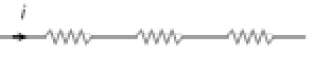II.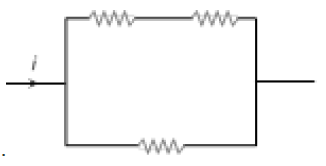III.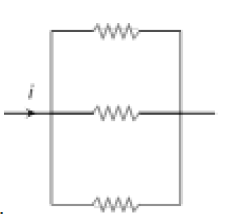IV.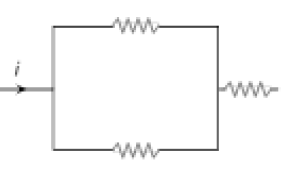• Option 1)

III < II < IV < I

• Option 2)

II < III < IV < I

• Option 3)

I < IV < III < II

• Option 4)

I < III < II < IV

As we learnt in  Series Grouping - Potential - Different Current - Same - wherein    & Parallel Grouping - Potential - Same Current - Different - wherein       P is maximum when R is     Order of power  iii <  ii < iv < i Option 1) III < II < IV < I This solution is correct Option 2) II < III < IV < I This solution is incorrect Option 3) I < IV < III < II This solution is incorrect Option...
Engineering
226 Views   |

The resistance of the filament of an electric bulb changes with temperature. If an electric bulb rated 220 volt and 100 watt is connected to (220×.8) volt sources, then the actual power would be

• Option 1)

100×0.8 watt

• Option 2)

100× (0.8)2 watt

• Option 3)

Between 100×0.8 watt and 100 watt

• Option 4)

Between 100× (0.8)2 watt and 100×0.8 watt

As we learnt in  Power dissipiated in external resistance - -     Applied Voltage=  maximum power =  Option 1) 100×0.8 watt This solution is incorrect Option 2) 100× (0.8)2 watt This solution is incorrect Option 3) Between 100×0.8 watt and 100 watt This solution is incorrect Option 4) Between 100× (0.8)2 watt and 100×0.8 watt This solution is correct
Engineering
683 Views   |

In the circuit shown in figure, the heat produced in 5 ohm resistance is 10 calories per second. The heat produced in 4 resistance is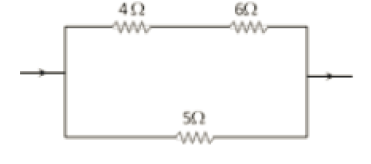• Option 1)

1 cal/sec

• Option 2)

2 cal/sec

• Option 3)

3 cal/sec

• Option 4)

4 cal/sec

As we learnt in Current through any resistance - - wherein   Let current through  be I1 and current through  be I2 . Then,  Option 1) 1 cal/sec This option is incorrect Option 2) 2 cal/sec This option is correct Option 3) 3 cal/sec This option is incorrect Option 4) 4 cal/sec This option is incorrect
Engineering
338 Views   |

In the circuit as shown in the figure, the heat produced by 6 ohm resistance due to current flowing in it is 60 calorie per second. The heat generated across 3 ohm resistance per second will be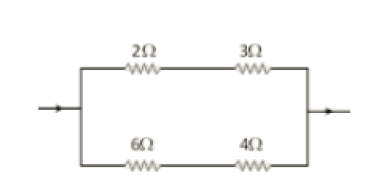• Option 1)

30 cal

• Option 2)

60 cal

• Option 3)

120 cal

• Option 4)

100 cal

As we learnt in  Power dissipiated in external resistance - -                                               Rate of Heat production          Option 1) 30 cal Incorrect Option 2) 60 cal Incorrect Option 3) 120 cal correct Option 4) 100 cal Incorrect
Engineering
92 Views   |

If a wire of resistance 20Ω is covered with ice and a voltage of 210 V is applied across the wire, then the rate of melting of ice is

• Option 1)

0.85g/s

• Option 2)

1.92g/s

• Option 3)

6.56g/s

• Option 4)

none of these

As we learnt in  Power dissipiated in external resistance - -    Rate of energy produces Energy required to melt one gm of ice = mL = 1   80  4.2 J = 336J/g      Rate of ice melting =  Option 1) 0.85g/s Incorrect Option 2) 1.92g/s Incorrect Option 3) 6.56g/s Correct Option 4) none of these Incorrect
Engineering
87 Views   |

An immersion heater is rated 836 watt. It should heat 1 litre of water from  to  in about

• Option 1)

200 sec

• Option 2)

150 sec

• Option 3)

100 sec

• Option 4)

120 sec

As we learnt in  Power dissipated in external resistance - -     Amount of energy required = m s  T                                            = 1 kg  (4.18 J/g)  30                                           = 4180  30J= 125400J Time required =                         Option 1) 200 sec Incorrect Option 2) 150 sec Correct Option 3) 100 sec Incorrect Option 4) 120 sec Incorrect
Engineering
119 Views   |

An electric kettle takes 4 A current at 220 V. How much time will it take to boil 1 kg of water from room temperature ? The temperature of boiling water is

• Option 1)

6.4 minutes

• Option 2)

6.3 minute

• Option 3)

12.6 minutes

• Option 4)

12.8 minutes

As we learnt in  Power dissipated in external resistance - -    Total energy required = m s T = 1000  1 cal/g  80 = 80000  4.2 J = 336000J   Option 1) 6.4 minutes Incorrect Option 2) 6.3 minute correct Option 3) 12.6 minutes Incorrect Option 4) 12.8 minutes Incorrect
Engineering
562 Views   |

A house is served by 220 V supply line in a circuit protected by a 9 ampere fuse. The maximum number of 60 W lamps in parallel that can be turned on, is

• Option 1)

44

• Option 2)

20

• Option 3)

22

• Option 4)

33

As we learnt in  Series Grouping - In series grouping anode of one cell is connected to cathode of other cell - wherein    Maximum power = 220   9W  number of Bulb  =  Option 1) 44 Incorrect option Option 2) 20 Incorrect option Option 3) 22 Incorrect option Option 4) 33 Correct option
Engineering
98 Views   |

A heater of 220 V heats a volume of water in 5 minute time. A heater of 110 V heats the same volume of water in

• Option 1)

5 minutes

• Option 2)

8 minutes

• Option 3)

10 minutes

• Option 4)

20 minutes

As we learnt in Power dissipiated in external resistance - -     Energy = P . t Initial energy = Final energy    Option 1) 5 minutes Incorrect Option Option 2) 8 minutes Incorrect Option Option 3) 10 minutes Incorrect Option Option 4) 20 minutes Correct Option
Engineering
393 Views   |

A 500 W heating unit is designed to operate from a 115 volt line. If the line voltage drops to 110 volt, the percentage drop in heat output will be

• Option 1)

10.2%

• Option 2)

8.1%

• Option 3)

8.6%

• Option 4)

7.6%

As we learnt in Power dissipiated in external resistance - -     Change                   Option 1) 10.2% Incorrect Option 2) 8.1% Incorrect  Option 3) 8.6% Correct  Option 4) 7.6% Incorrect
Exams
Articles
Questions